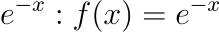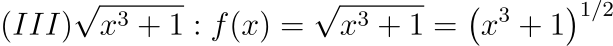Consider the functions

I. ${e}^{-x}$

II. ${x}^{2}-\mathrm{sin}x$

III. $\sqrt{{x}^{3}+1}$

Which of the above functions is/are increasing everywhere in [0,1]?

A.

III only

B.

II only

C.

II and III only

D.

I and III only

Solution:

To determine the nature of a function whether it is increasing or decreasing, we observe the first derivative of the function, i.e.For: (I)(By applying substitution and further solving)Comparing the functions I, II, III between the range [0, 1]: